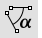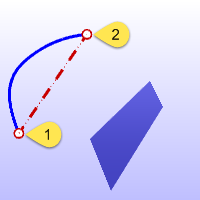# AngleAnalyze

Angle

The Angle command reports the angle between two sets of locations in degrees.

The angle is that between picked points that represent the endpoints of two lines.

#### Steps

1. Pick the start of an imaginary angle line.
Snap to existing geometry to establish the lines.
2. Pick the end of an imaginary angle line.3. Pick the start of a second imaginary angle line.
4. Pick the end of a second imaginary angle line.The angle between the imaginary lines is reported on the command line.
Command-line options

TwoObjects

Measures the angle between two planar surfaces or two lines.Type subcrv to select part of a curve as input.